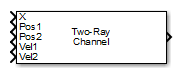# Two-Ray Channel

Two-ray channel environment

## Library

Environment and Target

`phasedenvlib`

•## Description

The Two-Ray Channel block propagates narrowband signals from one point in space to multiple points or from multiple points back to one point via both the direct path and the ground reflection path. The block models propagation time, free-space propagation loss, and Doppler shift. The block assumes that the propagation speed is much greater than the object's speed in which case the stop-and-hop model is valid.

## Parameters

Signal Propagation speed (m/s)

Specify the propagation speed of the signal, in meters per second, as a positive scalar. You can use the function `physconst` to specify the speed of light.

Signal carrier frequency (Hz)

Specify the carrier frequency of the signal in hertz of the narrowband signal as a positive scalar.

Specify atmospheric parameters

Select this check box to enable atmospheric attenuation modeling.

Temperature (degrees Celsius)

Ambient atmospheric temperature, specified as a real-valued scalar. Units are degrees Celsius. This parameter appears when you select the Specify atmospheric parameters check box. Units are degrees Celsius.

Dry air pressure (Pa)

Atmospheric dry air pressure, specified as a positive real-valued scalar. Units are Pascals (Pa). The value 101325 for this property corresponds to one standard atmosphere. This parameter appears when you select the Specify atmospheric parameters check box.

Water vapour density (g/m^3)

Atmospheric water vapor density, specified as a positive real-valued scalar. Units are gm/m3. This parameter appears when you select the Specify atmospheric parameters check box.

Liquid water density (g/m^3)

Liquid water density of fog or clouds, specified as a non-negative real-valued scalar. Units are gm/m3. Typical values for liquid water density are 0.05 for medium fog and 0.5 for thick fog. This parameter appears when you select the Specify atmospheric parameters check box.

Rain rate (mm/hr)

Rainfall rate, specified as a non-negative real-valued scalar. Units are in mm/hour. This parameter appears when you select the Specify atmospheric parameters check box.

Inherit sample rate

Select this check box to inherit the sample rate from upstream blocks. Otherwise, specify the sample rate using the Sample rate (Hz) parameter.

Sample rate (Hz)

Specify the signal sampling rate (in hertz) as a positive scalar. This parameter appears only when the Inherit sample rate parameter is not selected.

Ground reflection coefficient

Fraction of incident signal amplitude reflected towards receiver.

Combine two rays at output

Select this check box to coherently sum the direct-path and reflected-path signals at output. Clear the check box to keep the two rays separate.

Maximum one-way propagation distance (m)

The maximum distance between the signal origin and the destination, specified as a positive scalar. Units are in meters. Amplitudes of any signals that propagate beyond this distance will be set to zero.

Simulate using

Block simulation method, specified as `Interpreted Execution` or ```Code Generation```. If you want your block to use the MATLAB® interpreter, choose `Interpreted Execution`. If you want your block to run as compiled code, choose `Code Generation`. Compiled code requires time to compile but usually runs faster.

Interpreted execution is useful when you are developing and tuning a model. The block runs the underlying System object™ in MATLAB. You can change and execute your model quickly. When you are satisfied with your results, you can then run the block using ```Code Generation```. Long simulations run faster than they would in interpreted execution. You can run repeated executions without recompiling. However, if you change any block parameters, then the block automatically recompiles before execution.

When setting this parameter, you must take into account the overall model simulation mode. The table shows how the Simulate using parameter interacts with the overall simulation mode.

When the Simulink® model is in `Accelerator` mode, the block mode specified using Simulate using overrides the simulation mode.

Acceleration Modes

 Block Simulation Simulation Behavior `Normal` `Accelerator` `Rapid Accelerator` `Interpreted Execution` The block executes using the MATLAB interpreter. The block executes using the MATLAB interpreter. Creates a standalone executable from the model. `Code Generation` The block is compiled. All blocks in the model are compiled.

## Ports

Note

The block input and output ports correspond to the input and output parameters described in the `step` method of the underlying System object. See link at the bottom of this page.

PortDescriptionSupported Data Types
`X`

Input signal.

Double-precision floating point
`Pos1`

Signal source position.

Double-precision floating point
`Pos2`

Signal destination position.

Double-precision floating point
`Vel1`Signal source velocity.Double-precision floating point
`Vel2`

Signal destination velocity.

Double-precision floating point
`Out`

Propagated signal.

Double-precision floating point

## Algorithms

When the origin and destination are stationary relative to each other, the block output can be written as y(t) = x(t – τ)/L. The quantity τ is the delay and L is the propagation loss. The delay is computed from τ = R/c where R is the propagation distance and c is the propagation speed. The free space path loss is given by

`${L}_{fsp}=\frac{{\left(4\pi R\right)}^{2}}{{\lambda }^{2}},$`

where λ is the signal wavelength.

This formula assumes that the target is in the far-field of the transmitting element or array. In the near-field, the free-space path loss formula is not valid and can result in losses smaller than one, equivalent to a signal gain. For this reason, the loss is set to unity for range values, R ≤ λ/4π.

When there is relative motion between the origin and destination, the processing also introduces a frequency shift. This shift corresponds to the Doppler shift between the origin and destination. The frequency shift is v/λ for one-way propagation and 2v/λ for two-way propagation. The parameter v is the relative speed of the destination with respect to the origin.

## Version History

Introduced in R2021a